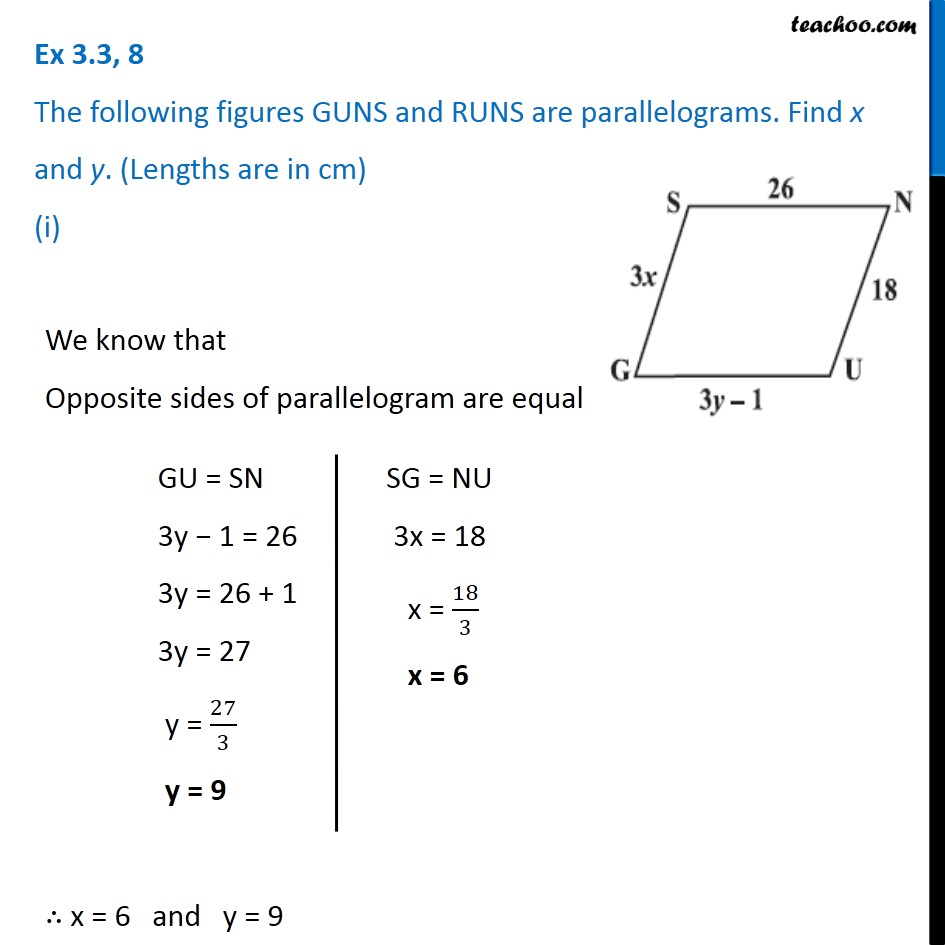Ex 3.3

Chapter 3 Class 8 Understanding Quadrilaterals
Serial order wise### Transcript

Ex 3.3, 8 The following figures GUNS and RUNS are parallelograms. Find x and y. (Lengths are in cm) (i) We know that Opposite sides of parallelogram are equal GU = SN 3y − 1 = 26 3y = 26 + 1 3y = 27 y = 27/3 y = 9 SG = NU 3x = 18 x = 18/3 x = 6 ∴ x = 6 and y = 9